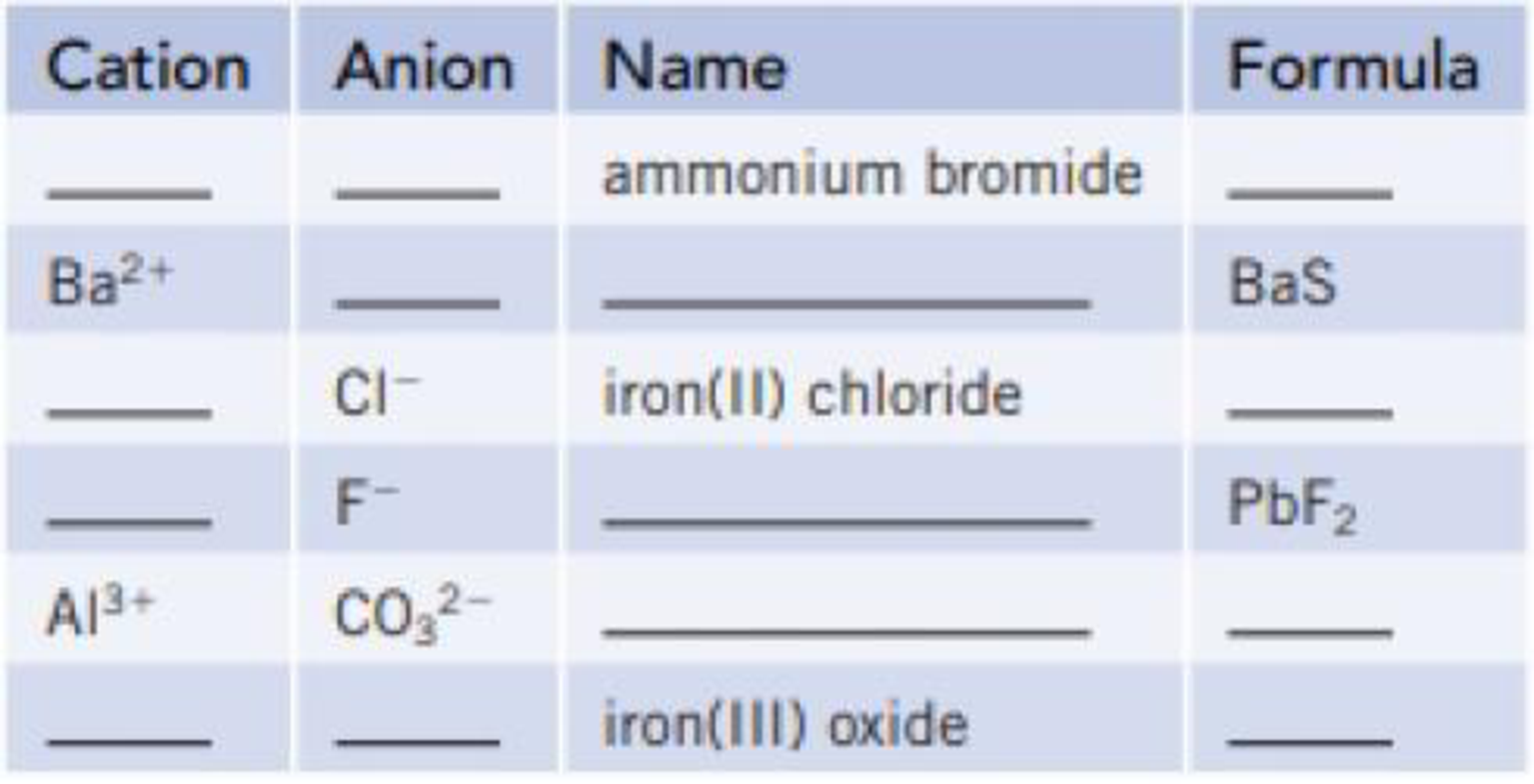Chapter 2, Problem 132GQ

Chapter
Section
Textbook Problem

Complete the table by placing symbols, formulas, and names in the blanks.Interpretation Introduction

Interpretation: The given table of cation, anion, name and formula for six compounds are needed to be completed.

Concept introduction:

Ionic compounds are formed by the combination of metal or NH4+ and nonmetals or metal and polyatomic ions. Metals have the positive charge (cation) and counterpart has the negative charge (anion).

Formula of a compound is the representation of atoms or polyatoms present in the compound by their respective symbol.

Naming of ions:

Positive ions (cations) are named as metal name, example: aluminum for aluminum cation.

But, for the transition metals the ionic charge also included by roman numerals with the naming of compound.

Negative ions (anions) are named by adding ‘ide’ to the stem of the nonmetal element, from which the anion is formed, example: chloride, bromide, etc.

Explanation

In ammonium bromide, ammonium is the cation and bromine ion is the anion. The charges of ions are +1 and -1 respectively, so they are balanced. Hence, the formula of the ammonium bromide is NH4Br.

In BaS, barium and sulfur ions are present. Barium is the cation and sulfide is the anion.

Hence, the name of BaS is barium sulfide.

In iron chloride, iron is the cation and chlorine ion is the anion. The charges of iron ion is +2 and chlorine ion is -1, so they are balanced with 1:2 ratio (cation:anion). Hence, the formula of the iron chloride is FeCl2.

In PbF2, lead and fluorine ions are present. Lead is the cation and fluoride is the anion.

Hence, the name of PbF2 is Lead fluoride

Still sussing out bartleby?

Check out a sample textbook solution.

See a sample solution

The Solution to Your Study Problems

Bartleby provides explanations to thousands of textbook problems written by our experts, many with advanced degrees!

Get Started

Find more solutions based on key concepts